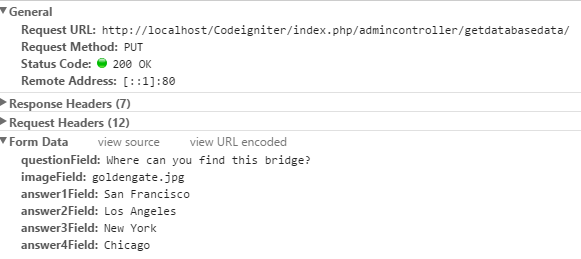# Rest Api PUT method not receiving values

I have this code in Ajax/Jquery, where I am trying to request a PUT method to my API. In my code I am trying to send the data that has been updated in my text fields. This data should be received by the API and send it to the Model it should be used to update a records in my database based on the ID recived. For some reason, my REST API in the controller is not receiving the values from my text fields.

Code where I am sending the data:

``````\$("#edit").on("click", function() {
var updatedData ={ question: \$('#questionField').val(),
image: \$('#imageField').val(),
};
\$.ajax({
type: 'PUT',
dataType: 'json',
data: updatedData,

success: function(updatedQuestion)
{
JSON.stringify(updatedQuestion);
if(updatedQuestion.ok == 1) {
}
else{
}
}
});
return false;
});
``````
``````Code for my REST API where data should be received:
``````
``````    else if (\$this->input->server('REQUEST_METHOD') == 'PUT' )
{
\$id = \$this->input->post('idField');
\$question = \$this->input->post('questionField');
\$image = \$this->input->post('imageField');

if(\$question != '' && \$image != '' && \$answer1 != '' && \$answer2 != '' && \$answer3 != '' && \$answer4 != ''){
echo json_encode(array('ok' => 1));
}
else{
echo json_encode(array('ok' => \$question));
}

}
``````

This what I am getting in the chrome network tab:At the moment I am trying to alert to see if the data is passed, but I just get NULL. Any idea why? Thanks.

The chrome network tab shows that the data is being sent correctly. In the php does post need to be put ?

``````\$this->input->put('idField');
``````

Is the API returning JSON with text/html headers instead of application/json ?

I tried POST and PUT but everything returns null…

I don’t understand… Sorry

If the AJAX is expecting to get JSON but is getting text (albeit formatted as JSON) it will not be able to parse it hence a null.

``````header("Content-type: application/json");
``````

to the beginning of the file that returns it.

Ok. So basically I have this function getdatabasedata() where I have:

``````        if(\$this->input->server('REQUEST_METHOD') == 'GET')
{ do something }
else if (\$this->input->server('REQUEST_METHOD') == 'POST')
{ do something }
else if (\$this->input->server('REQUEST_METHOD') == 'PUT' )
{
\$question = \$this->input->post('questionField');
echo json_encode(array('ok' => \$question));
}
``````

I am just trying to send it back to see if the success: function can alert the sent data. Just to test.
I am having somehow exactly the same code for the POST, and it does create a new record in the database. I don’t know why the PUT is not working.

This is the code for my POST which should be mostly the same as the PUT.

``````else if (\$this->input->server('REQUEST_METHOD') == 'POST')
{
\$question = \$this->input->post('question');
\$image = \$this->input->post('image');

if(\$question != '' && \$image != '' && \$answer1 != '' && \$answer2 != '' && \$answer3 != '' && \$answer4 != ''){
echo json_encode(array('ok' => 1));
}
else{
echo json_encode(array('ok' => 0));
}
}
``````

What I am really not understanding how the data is passed. For example this part: `\$question = \$this->input->post('question');`
This is getting the data straight from the text field? or from here:

``````        var question ={ question: \$('#question').val(),
image: \$('#image').val(),